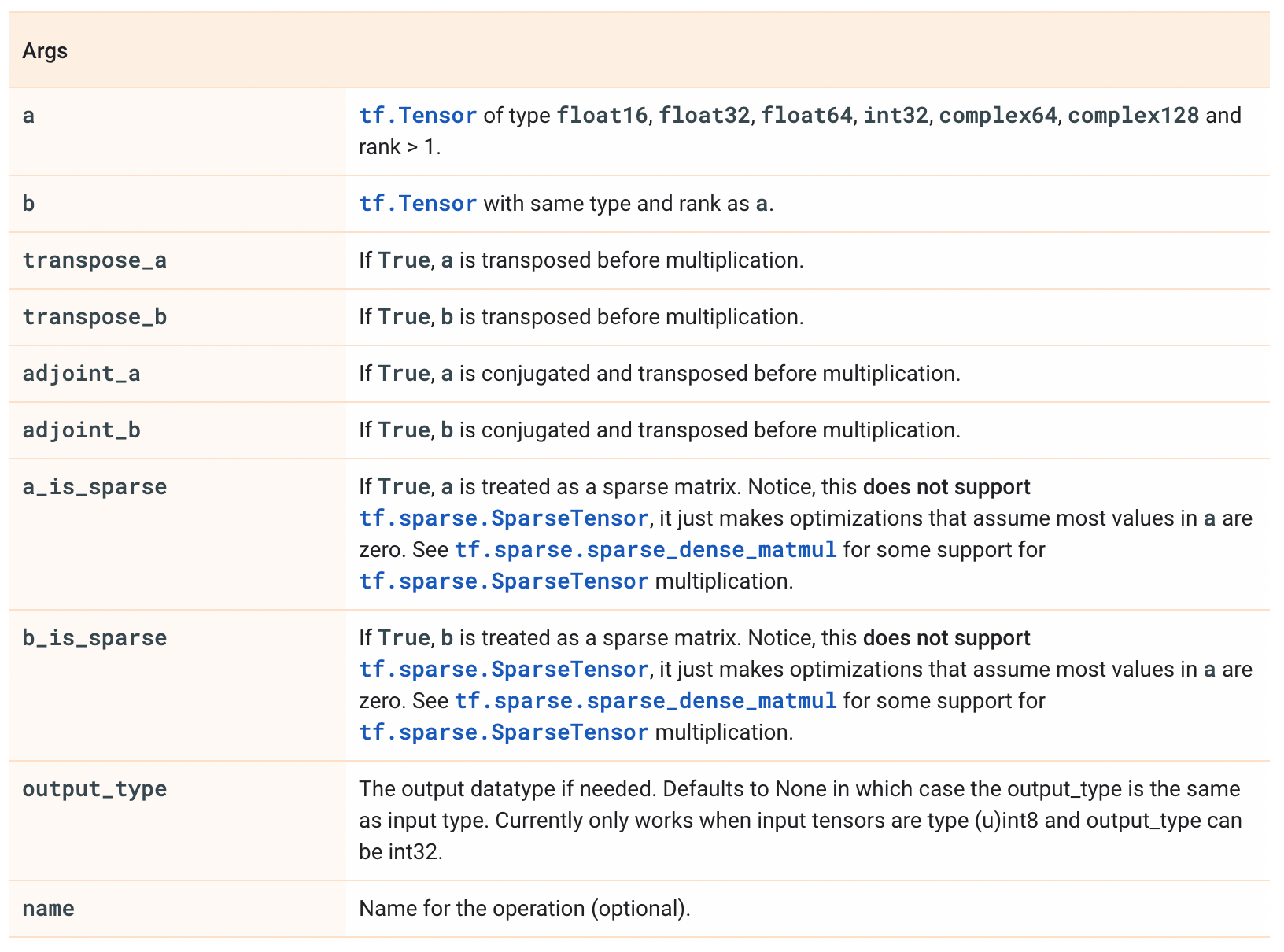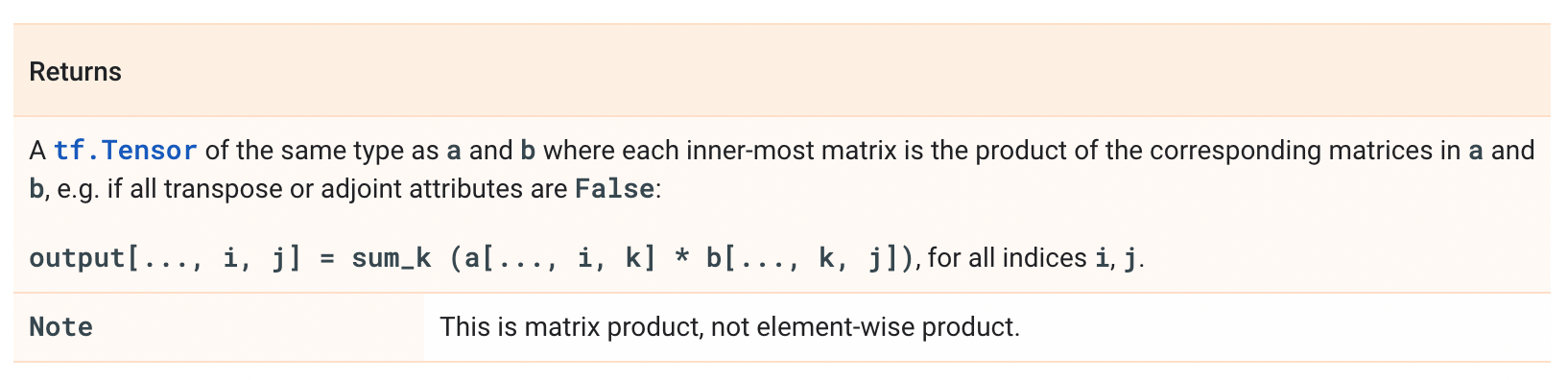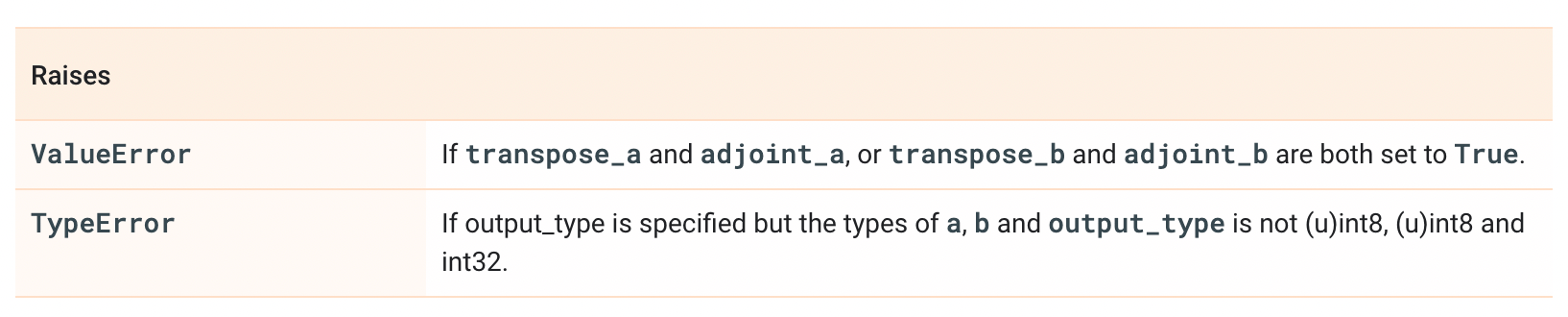×

Search anything:

# MatMul in TensorFlow

#### Machine Learning (ML) TensorFlowGet this book -> Problems on Array: For Interviews and Competitive Programming

In this article, we have explored MatMul operation in TensorFlow (tf.linalg.matmul()) and have presented a sample TensorFlow Python code performing MatMul (Matrix Multiplication).

1. Introduction
2. tf.linalg.matmul() + TF code for MatMul
3. Glossary

# Introduction

Machine learning, and for that matter artificial intelligence, uses a wide range of data to design models for a variation of tasks. This variation in the use of these models means the data types vary as well. In most scientific applications of A.I, vectors(matrices) are used to express quantities in differnt dimensions, quantities with different properties(i.e: direction and magnitude etc), etc. In non-scientific applications of A.I, vectors(matrices) are used to show different qualities of one form of data, that means every column/row has a specific meaning.

The tensorflow library can be used in many applications of Machine Learning. Part of its usefullness is also it's flexibility, to work with different data types using tensors. A tensor is a generalization of vectors and matrices to potentially higher dimensions. Tensorflow represents tensors as n-dimensional arrays of specified data types. So a single scaler can be represented as a 1x1 matrix.

As tensors are n-dimesional matrices, tensorflow hence has a variety of matrix operations, example matrix multiplication which uses the MatMul operation in the linalg class of the tensorflow library. In this article we will discuss the features supported by the tensorflow.linalg.matmul operation with tensorflow.

# tf.linalg.matmul() + TF code for MatMul

The tf.linalg.matmul() function is used for 2 dimention matrix multiplication. The following is the general definition of the function:

Following any manipulations, tensors among other things must be matrices with a rank of 2, which means the maximum number of its linearly independent vectors.The tensors must also be compatible matrices and specify valid matrix multiplication dimensions, which means the inner 2 dimensions must be equal, and any further outer dimensions specify matching batch size.

Matrices a,b defined in tf.linalg.matmul(a,b) must also be of the same data type. The data type can be defined using the dtype attribute and the list of supported data types for tensors are: bfloat16, float16, float32, float64, int32, int64, complex64, complex128, the default is int32. See below for some example code illustrating this...

``````import tensorflow as tf

a = tf.constant([1, 2, 3, 4, 5, 6], shape=[2, 3]) #returns a 2x3 int32 matrix
b = tf.constant([7, 8, 9, 10, 11, 12], shape = [3, 2]) #returns a 3x2 int32 matrix
c = tf.constant([13, 14, 15, 16, 17, 18], shape=[3, 2]), dtype=tf.float32) #returns a 3x2 float32 matrix
d = tf.constant([19, 20, 21, 22, 23, 24, 25, 26], shape=[2, 4]) #returns a 2x4 int32 matrix
e = tf.linalg.matmul(a, b) #returns a valid response
f = tf.linalg.matmul(a,d) #invalid response because the matrices aren't compatiple
g = tf. linalg.matmul(a,c) #invalid response because matrices aren't of the same datatype
h = tf.linalg.matmul(b,d) #returns a valid response but note that there are mismatching batch sizes.
# which are the outer layers

# for all of the matmul operations use the following sample code and run it to see the results on the console

# try:
# e = tf.linalg.matmul(a, b)

# print(e)
# except Exception as ee:
# print(ee)
# do this for f, g, and h and read the errors on the console
``````

Either matrix can be transposed or adjointed(conjugated then transposed) on the fly by setting the corresponding flag of the matrix in question to True. These are False by default. Also, if one or both of the matrices contain a lot of zeros, a more efficient multiplication algorithm can be used by changing the corresponding a_is_sparse or b_is_sparse flag from False to True. This optimization is only available for plain matrices (rank-2 tensors) with datatypes bfloat16 or float32.

i.e.:(tf.linalg.matmul(a, b, transpose_a=True, transpose_b=False, adjoint_a=False, adjoint_b=True). Now lets see what the individual flags do to a matrix. Note that none of our matrices have complex numbers, hence adjoint will not be necessary.

``````a = tf.constant([1, 2, 3, 4, 5, 6], shape=[2, 3])
b = tf.constant([7, 8, 9, 10, 11, 12], shape = [3, 2])
c = tf.constant([13, 14, 15, 16, 17, 18], shape=[3, 2]), dtype=tf.float32)
d = tf.constant([19, 20, 21, 22, 23, 24, 25, 26], shape=[2, 4])
e = tf.linalg.matmul(a, b, name='a,b matrix multiplication') # returns a valid response
f = tf.linalg.matmul(a,d, transpose_a=True, output_type=float, name='transpose a to make matrices compatible') #a is now a 3x2 matrix making them now compatible
``````

Input and Output:

``````#-----------------
# Input Tensors
#-----------------
#Matrx a:
#tf.Tensor(
#[[1 2 3]
# [4 5 6]], shape=(2, 3), dtype=int32)
#
#Matrx b:
#tf.Tensor(
#[[ 7  8]
# [ 9 10]
# [11 12]], shape=(3, 2), dtype=int32)
#
#Matrx c:
#tf.Tensor(
#[[13. 14.]
# [15. 16.]
# [17. 18.]], shape=(3, 2), dtype=float32)
#
#Matrx d:
#tf.Tensor(
#[[19 20 21 22]
# [23 24 25 26]], shape=(2, 4), dtype=int32)
#
#----------------
# Output Tensors
#----------------
#e = axb:
#tf.Tensor(
#[[ 58  64]
# [139 154]], shape=(2, 2), dtype=int32)
#
#f = a(transposed)xd:
#tf.Tensor(
#[[111 116 121 126]
# [153 160 167 174]
# [195 204 213 222]], shape=(3, 4), dtype=int32)
#
#h = bxd:
#tf.Tensor(
#[[317 332 347 362]
# [401 420 439 458]
# [485 508 531 554]], shape=(3, 4), dtype=int32)
``````

If you notice in the code above, I have also named the operations and changed the data type of the output using the name, output_type flags.

Side Note:
A sparse matrix is one in which the majority of the elements are zero values. The sparsity of a matrix is defined by, number of zero elements / total number of elements. Hence, a matrix is sparse if the sparsity is greater than 50%. The interest in sparsity arises because its exploitation can lead to enormous computational savings(basically, computational arithmetic is much easier with 0) and because many large matrix problems that occur in practice are sparse. If you have such a matrix, you can set the sparsity as true(i.e.: tf.linalg.matmul(a, b, a_is_sparse=True, b_is_sparse=False).# Glossary

Transposing a matrix means changing its rows to columns and vice versa. Hence, transposing a matrix changes its size from rxc to cxr(i.e: a 2x3 matrix after transposing will be 3x2).

Adjointing a matrix is the process of conjugating a matrix before transposing. Conjugating a matrix is useful for matrices that have elements that have 2 dimensions, i.e: complex numbers(3 + 2i). The conjugate of a number is one with the same real part but the opposite imaginary part. So the conjugate of 3 + 2i is 3 - 2i. After conjugating every element in the matrix (i.e,: finding the conjugate of a matrix), then you transpose the matrix to complete the adjointing process.

You can multiply a scalar with a matrix to produce a different matrix. This is done by simply multiplying each element by the scalar. For example; a = [[1 2 3], [4 5 6], [7 8 9]], then k.a = [[k 2k 3k], [4k 5k 6k], [7k 8k 9k]].

A different kind of matrix multiplication multiplies 2 or more matrices. This is done by multiplying each element of each column of one matrix by each element of each row of another matrix. This means, the number of columns of the first matrix should be equal to the number of rows of the second matrix, making them compatible. This means an n x m matrix can only be multiplied with a compatible m x y matrix, resulting in a n x y matrix. For example; if matrix_a is a 2 x 3 matrix and matrix_b is a 3 x 10 matrix then matrix_c which is matrix_a x matrix_b will be a 2 x 10 matrix. Note that, as matrices represent vectors a x b is not always equal to b x a. For example; matrix_a(2 x 3) can be multiplied by matrix_b(3 x 10), but matrix_b cannot be multiplied by matrix_a in the opposite order because the number of columns in the first matrix is now not equal to the number of rows in the second i.e.: 10 to 2). However, if you transposed matrix_c(10 x 2) then you can now do matrix_b x matrix_c(transpose), or matrix_c(transposed) x matrix_a, or better yet, matrix_c x matrix_a(transposed). Even if switching the order didn't affect ompatibility, the resulting matrix after multiplication will not always be the same.